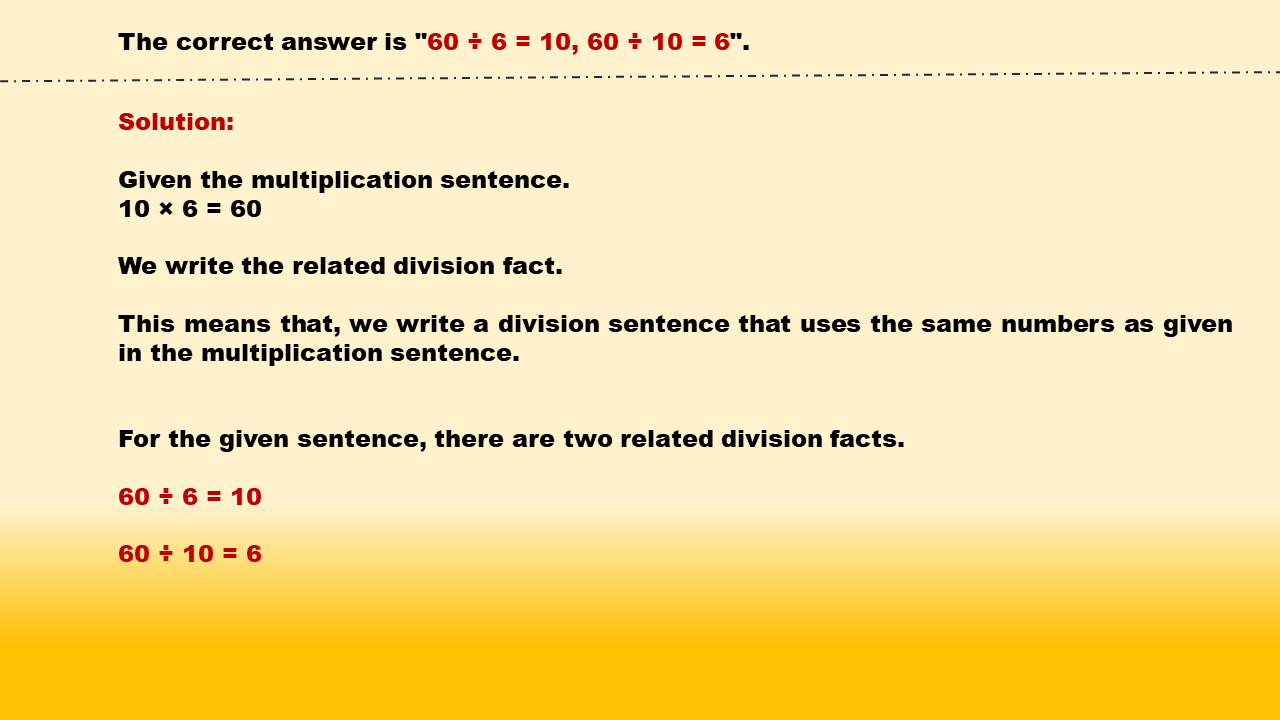1
visibility

Identify related division facts for 10 × 6 = 60.

• 70 ÷ 7 = 10, 70 ÷ 10 = 7

• 60 ÷ 6 = 10, 60 ÷ 10 = 6

• 50 ÷ 5 = 10, 50 ÷ 10 = 5

• 40 ÷ 4 = 10, 40 ÷ 10 = 4